Get Free NCERT Solutions for Class 10 Maths Chapter 2 Ex 2.2 PDF. Polynomials Class 10 Maths NCERT Solutions are extremely helpful while doing homework. Exercise 2.2 Class 10 Maths NCERT Solutions were prepared by Experienced LearnCBSE.in Teachers. Detailed answers of all the questions in Chapter 1 maths class 10 Polynomials Exercise 2.2 provided in NCERT TextBook.

Topics and Sub Topics in Class 10 Maths Chapter 2 Polynomials:

 Section Name Topic Name 2 Polynomials 2.1 Introduction 2.2 Geometrical Meaning Of The Zeroes Of A Polynomial 2.3 Relationship Between Zeroes And Coefficients Of A Polynomial 2.4 Division Algorithm For Polynomials 2.5 Polynomials 2.6 Summary

You can also download the free PDF of  Ex 2.2 Class 10 Polynomials NCERT Solutions or save the solution images and take the print out to keep it handy for your exam preparation.

 Board CBSE Textbook NCERT Class Class 10 Subject Maths Chapter Chapter 2 Chapter Name Polynomials Exercise Ex 2.2 Number of Questions Solved 2 Category NCERT Solutions

## NCERT Solutions For Class 10 Maths Chapter 2 Ex 2.2

NCERT Solutions for Class 10 Maths Chapter 2 Polynomials Ex 2.2 are part of NCERT Solutions for Class 10 Maths. Here we have given Maths NCERT Solutions Class 10 Chapter 2 Polynomials Exercise 2.2.

A simple online Degree and Leading Coefficient Calculator which is a user-friendly tool.

Ex 2.2 Class 10 Maths Question 1.
Find the zeroes of the following quadratic polynomials and verify the relationship between the zeroes and their coefficients:
(i) x2 – 2x – 8
(ii) 4s2 – 4s + 1
(iii) 6x2 – 3 – 7x
(iv) 4u2 + 8u
(v) t2 – 15
(vi) 3x2 – x – 4
Solution: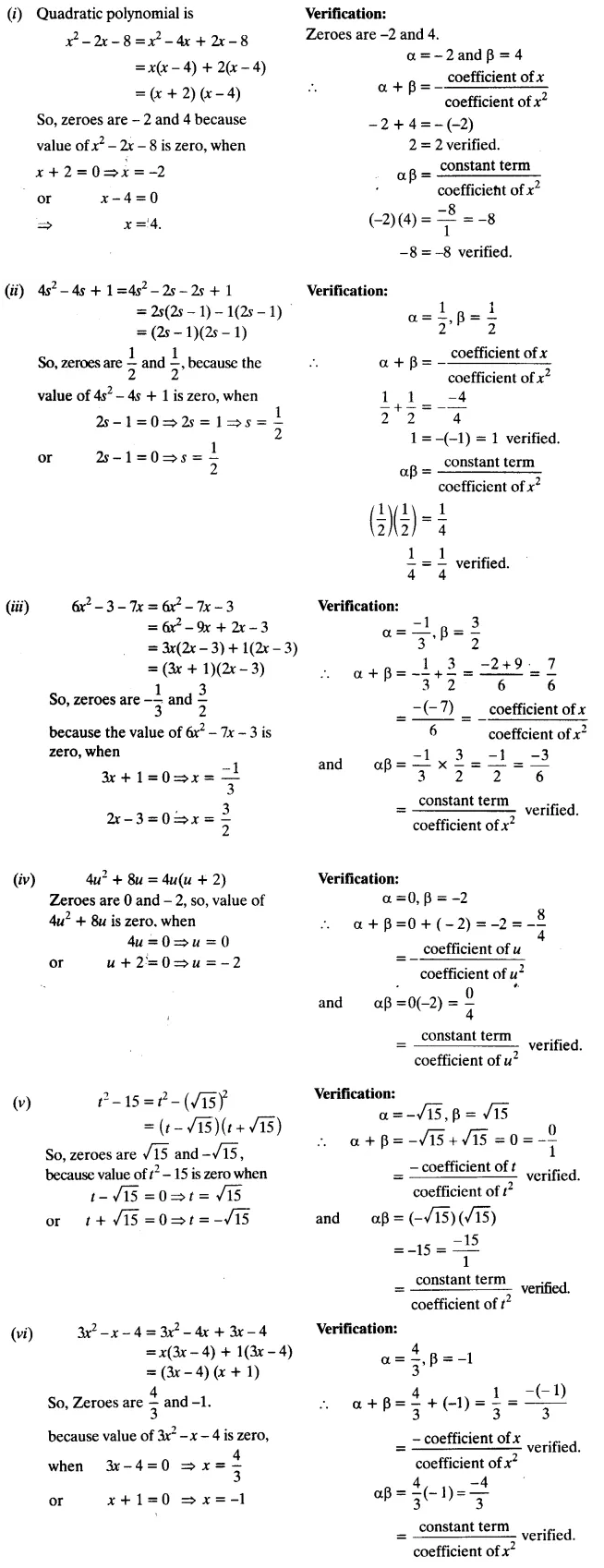Ex 2.2 Class 10 Maths Question 2.
Find a quadratic polynomial each with the given numbers as the sum and product of zeroes respectively: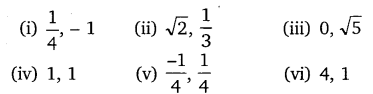Solution:### NCERT Solutions for Class 10 Maths Chapter 2 Polynomial (Hindi Medium) Ex 2.2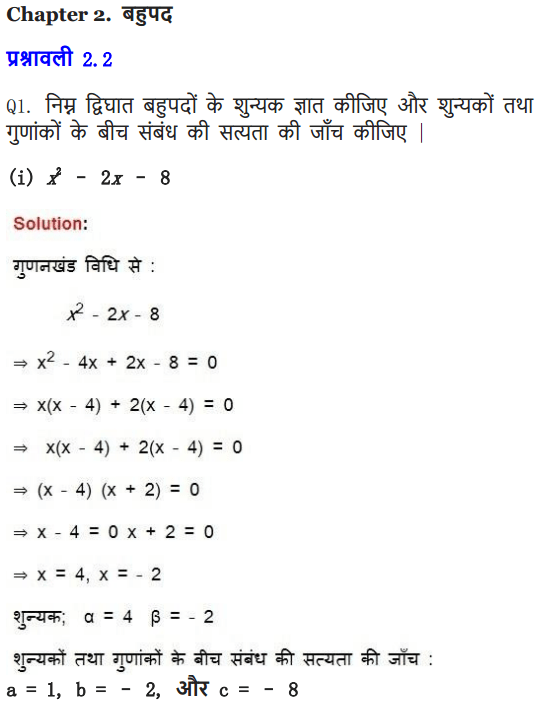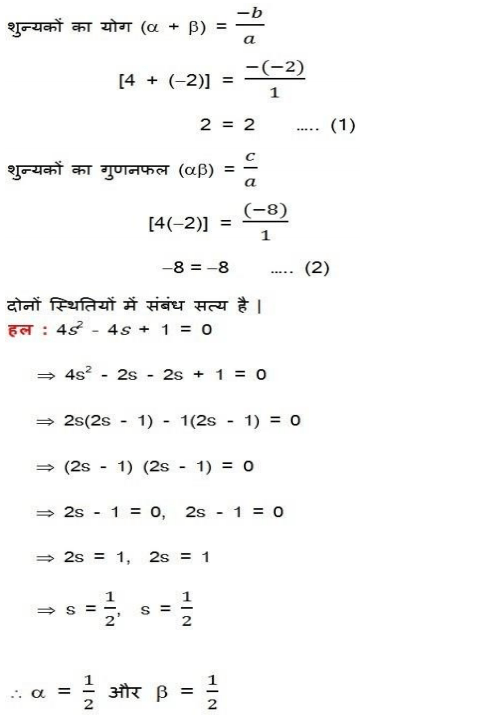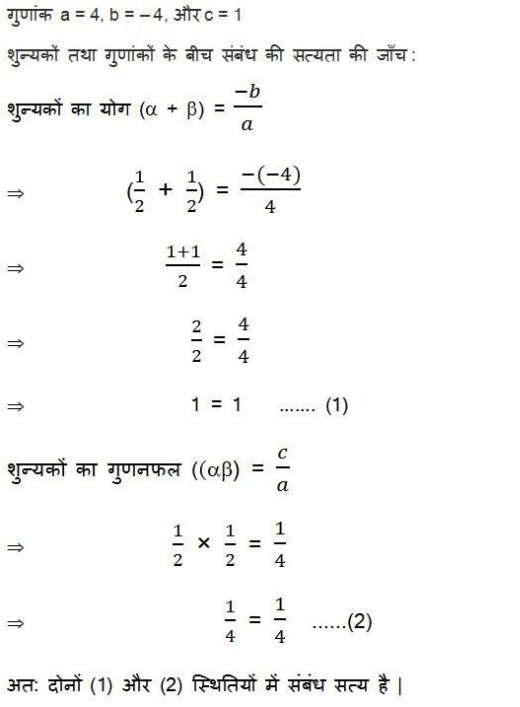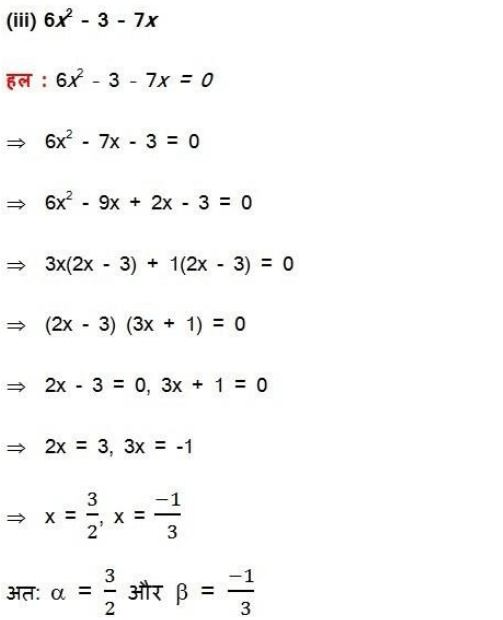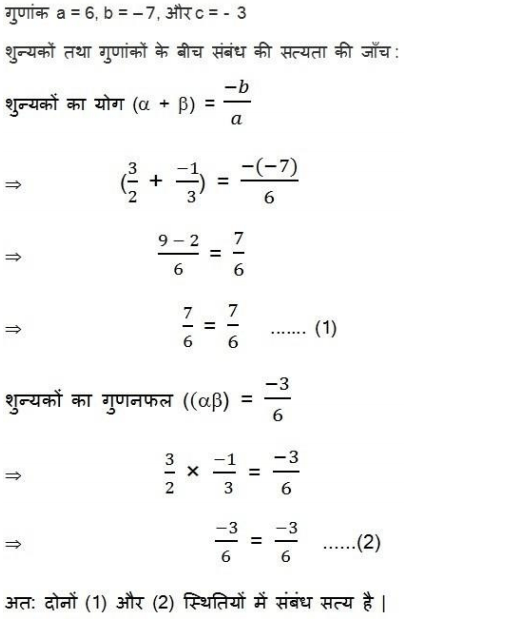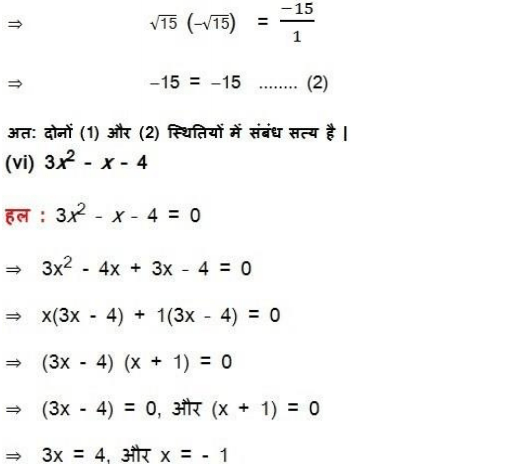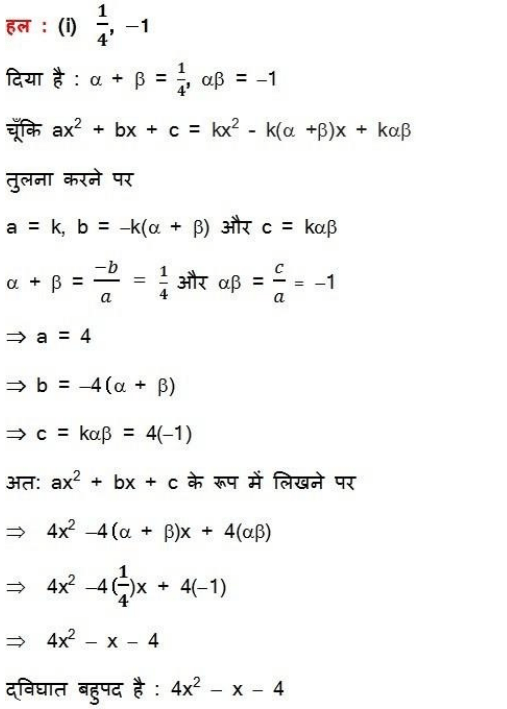We hope the NCERT Solutions for Class 10 Maths Chapter 2 Polynomials Ex 2.2, help you. If you have any query regarding Maths NCERT Solutions Chapter 2 Polynomials Exercise 2.2, drop a comment below and we will get back to you at the earliest.